# Tangent line to graph

## Summary and examples

We want to find an equation for the tangent line at a pointof the graph of a function.
Before we go into this in more detail, we will first discuss the following general case: what is the equation of the line through a point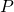with coordinates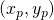with slope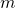.
The general equation of a line is: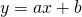Because the slope is equal tois, we have: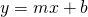This line has to go through the point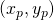, thus we write: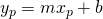from which it follows: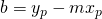Now the formula for the line is:or: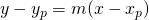It is most convenient to just remember this formula and this is not difficult given the simple, symmetric shape.

Now we return to the equation of a tangent line at a point of the graph of the function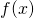. When we want to determine the tangent line at the point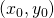of the graph of the function, the slope can be found by: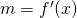and thus de formula for the tangent line is: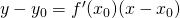##### Example 1

Determine the tangent line at the point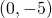of the graph of the function: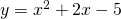First we calculate the derivative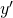of the function: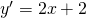In the pointwe have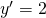and this is the slope of the tangent line at.

According to the formula given above the equation of the tangent line is: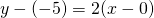or: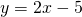##### Example 2

Where does the tangent line at the point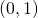of the graph of the function: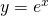intersect the-axis.

First we determine the equation of the tangent line in the point.

The derivative of the function is: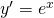In the pointthe derivative has the value, so the slope of the tangent line is. The tangent line goes through the pointand thus the equation of the tangent line is: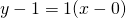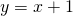The tangent line intersects the-axis in the point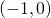.

##### Example 3

Given the function: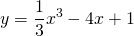Determine the equation of the tangent line to the graph of this function, parallel to the line: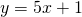The slope of the tangent line to the graph of the given function is found by differentiation: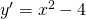In a point  with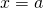the slope of the tangent line is: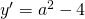The line is parallel to the given line, so: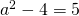or: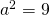From this we may conclude: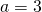or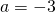The corresponding tangent points are: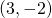or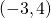The tangent lines through these points are, respectively: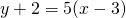or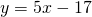or: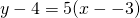or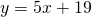0